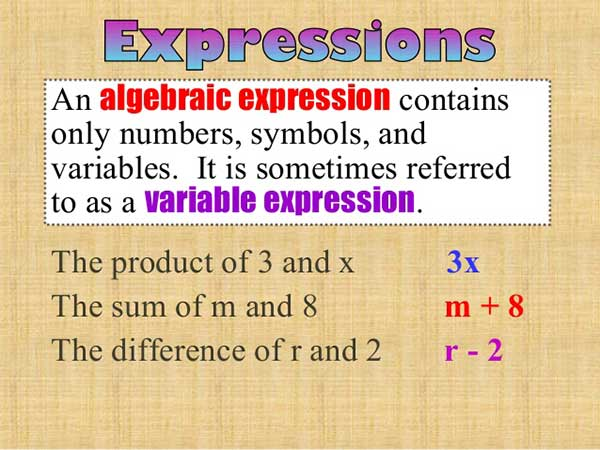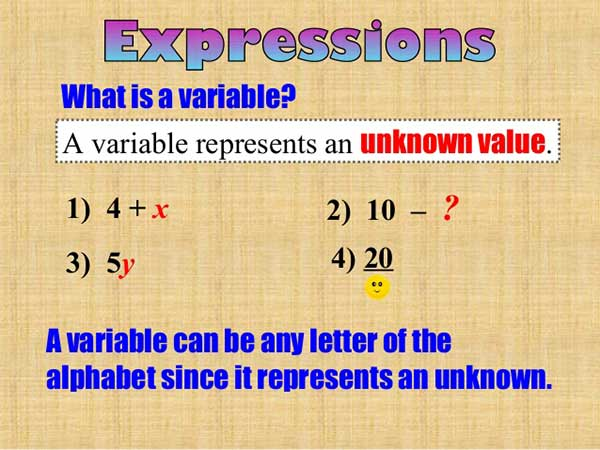Algebraic expressions  by  Joab Otieno

6

What is an algebraic expression?What is a variable?1/4w + 3 = 5

#### step 1

Subtract 3 from both sides
1/4w+3-3=5-3
1/4w=2

#### step 2

Multiply both sides by 4
4×1/4w=2×4
W=8

3(x+2)=7

#### step 1

Remove the brackets ( ) by multiplying 3 by x and 3 by 2
3x+6=7

#### step 2

Subtract 6 from both sides
3x+6-6=7-6
3x=1

#### step 3

Divide both sides by 3
3/3x=1/3
X= 1/3

#### Exercise

Work out the following
1) 1/3x+8=11
2) 1/5m-3=9
3) 1/7k+8=14
4) 3(n+2n)=18
5) 5(3a+1) =35